|

# 一键开启恒温淋浴体验 高宝恒温花洒测评

下班很疲惫，洗澡很重要，那么花洒就成为解决问题的关键。本次小编就给大家推荐一款厉害的花洒——高宝恒温花洒，它既要有靓丽的外观，又要满足环保节能的需要，还拥有和苹果手机一样简单便捷的一键操控按钮。下面，就请小编一起来实地测试这款产品吧。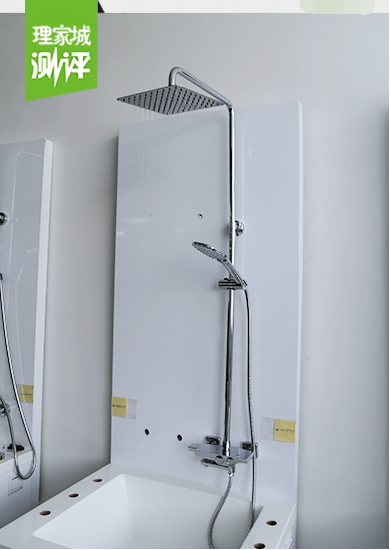高宝恒温花洒由顶喷、手持花洒、下水龙头组成，整体设计简约时尚，是现代简约卫浴风格的首选。在材质上，高宝恒温花洒精选国际标准黄铜材质，不含杂质，含铅量低，水质更加纯净环保；在工艺上，高宝首创17道除铅技术和工艺，并通过美国NSF认证，让消费者的淋浴用水也远离铅毒的危害。

38°恒温 不再忽冷忽热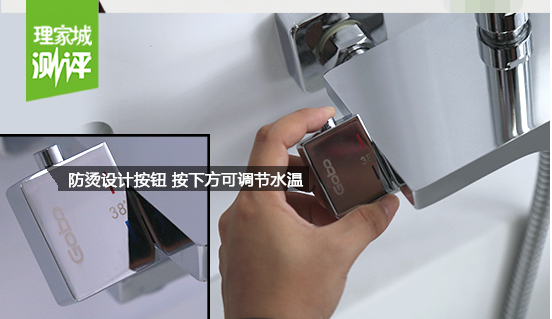顾名思义，高宝恒温花洒预设38℃舒适安全水温，与此同时，这款恒温花洒的最高安全温度设定为49℃，杜绝消费者在淋浴的过程中会被高温烫伤。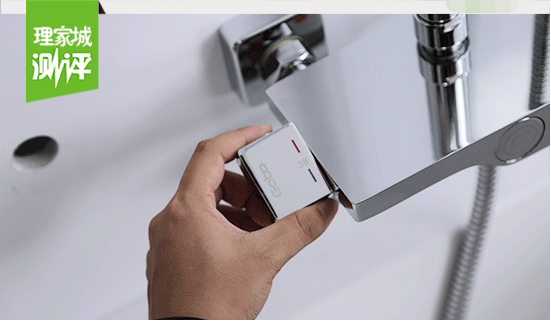普通花洒在淋浴时，需要打开开关用手去试出水的温度，在出水温度不合适时，要扳动手柄进行调节，有时我们可能要进行反复的调节，才能找到合适的温度。而高宝恒温花洒，只需要在淋浴之前拧到自己想要的温度刻线，无需等待，出水温度就是舒适的淋浴温度。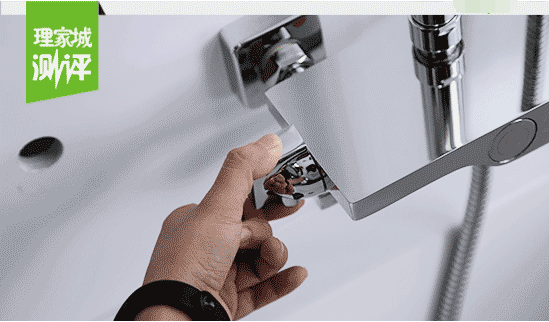法国进口阀芯让高宝花洒恒温性能更稳定，恒定温度不超过2℃，使用寿命可达50万次。小编现场测试：高宝恒温花洒带有误操作按钮设计，调节38℃以上温度必须要按下按钮才可以扭动调温，防止家中老人小孩因误操作而烫伤。

时尚百搭外观 满足多种装修搭配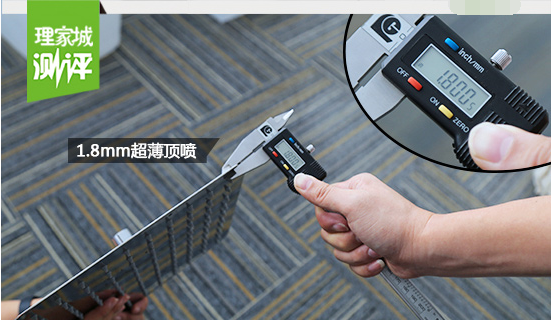有别于传统厚重型花洒，高宝恒温花洒显得细致和用心。花洒顶喷采用超薄方正外观设计，小编现场用卡尺测量，仅有1.8mm。超薄顶喷设计避免了传统花洒头内残留水过多的现象，小编现场测试，高宝花洒，开关关掉后3秒，花洒滴水彻底停止。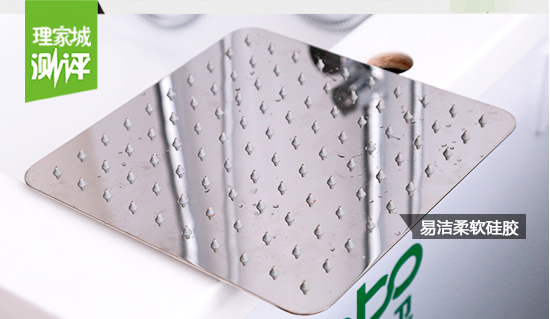高宝恒温花洒顶喷及手持花洒的出水孔均采用耐腐蚀、耐老化的易洁柔软硅胶。易洁硅胶让日常清洁维护变得简单便捷，只需要轻轻擦拭富有弹性的硅胶喷嘴即可清除水垢。北方的小伙伴们再也不用担心“水质硬易堵塞”的问题。好产品自己会说话。高宝恒温花洒表面使用三层以上精密电镀工艺，表面光亮如镜。小编现场测试，花洒可以当作化妆镜用。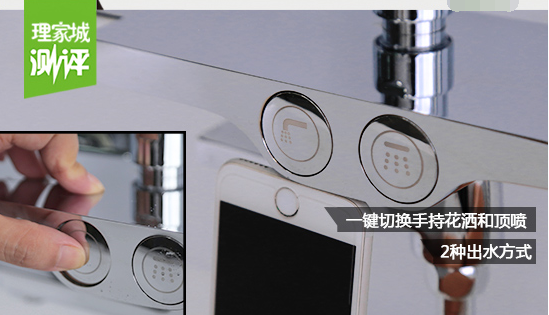想享受高效、便捷的淋浴体验？高宝恒温花洒可以满足你。整个花洒的开关使用一键按钮开关。只需轻轻一按，便可自由切换顶喷、手持花洒之间的出水。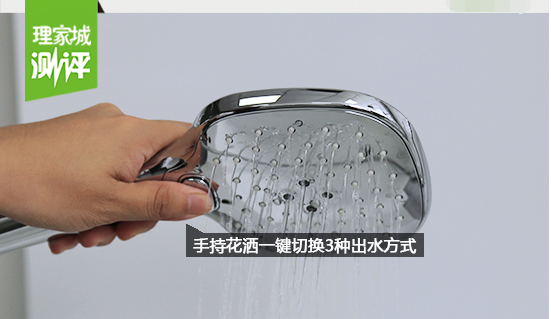手持花洒一键切换3种出水方式，三种出水方式给消费者带来不同的淋浴享受。

柔和、流畅、亲肤 多种出水方式体验

顶喷出水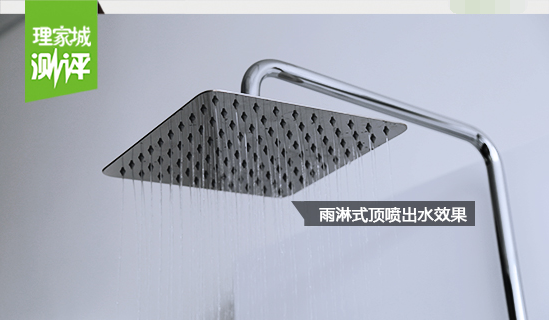顶喷雨淋式出水：高宝恒温花洒采用领先的空气混入技术，每一滴水都包含了30%的空气，畅享舒适的沐浴享受的同时节水30%。小编用手亲自体验过高宝顶喷花洒出水柔和度，流畅、饱满的水珠，连绵不断，打在手上无刺痛感。

手持花洒出水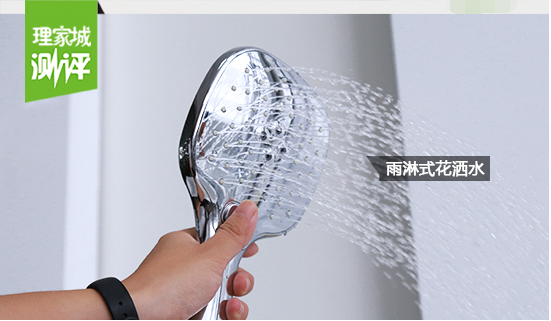雨淋式出水方式：雨淋式是消费者日常使用频率最高出水方式。柔和的水珠，可贴近身体的任何部位，洗净一身尘埃与疲惫，达到身心放松的状态。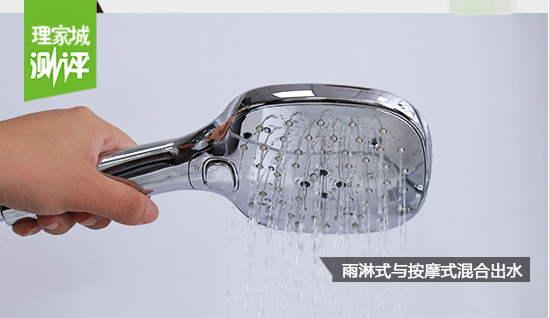混合出水方式：如果你想按摩，又想淋浴，那么混合式出水是你最完美的选择。中间的按摩水柱可刺激穴位，周围的水柱可洁净身体，舒缓与清洁身体一举两得。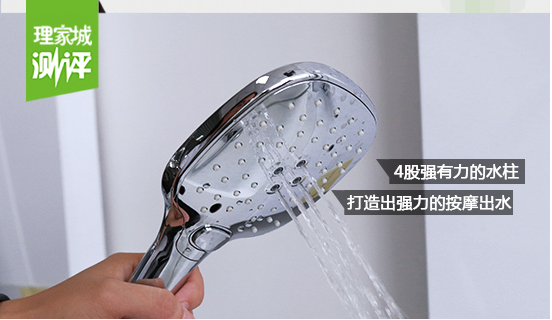空气按摩式出水：按摩式出水方式适用于所有肌肤。4股强有力的水柱可集中“水力”按摩身体。尤其是肩部劳损过度的上班族，可大胆使用按摩式出水按摩肩部，释放压力、恢复体能。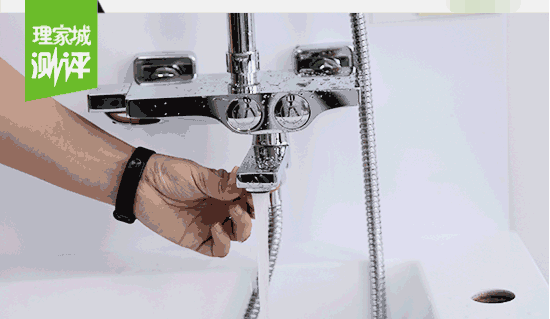下水龙头出水：为了方便用户日常取水，节省空间，高宝恒温花洒还设置可90°旋转下出水龙头。旋转龙头同时具有开关下水龙头的功能，扭出来即为出水，扭近墙体即为关闭。同时，下水龙头还内置节水起泡器，有效过滤水中杂质，水流舒缓柔顺，防止溅水。

人性化设计 带置物平台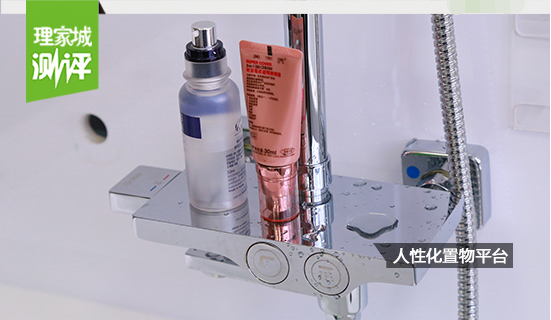花洒的开关设计上还加入了置物架，洗面奶、沐浴露、香皂等就放置在眼前，想用随手可取，不需要离开花洒。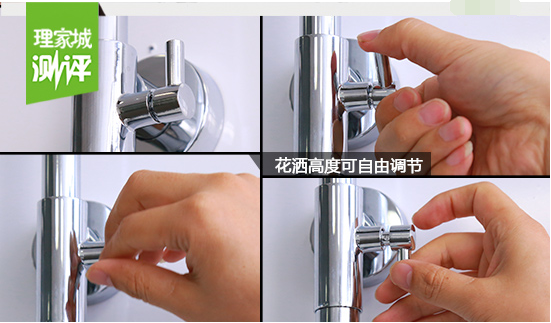在不锈钢淋浴柱上，设置了整体升降功能，消费者可根据一家老小的身高自由调节高度。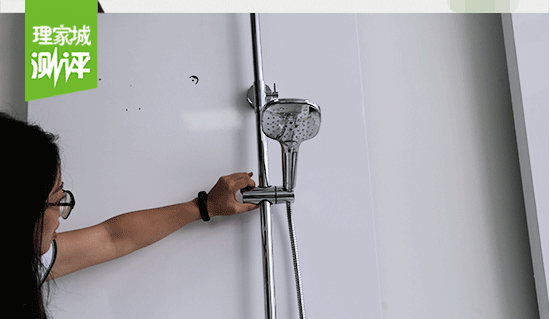与此同时，手持花洒放置架上，还设有自由调节按钮，一家男女老少都能可以找到自己的位置。小编现场测试，上下自由滑动可达48cm。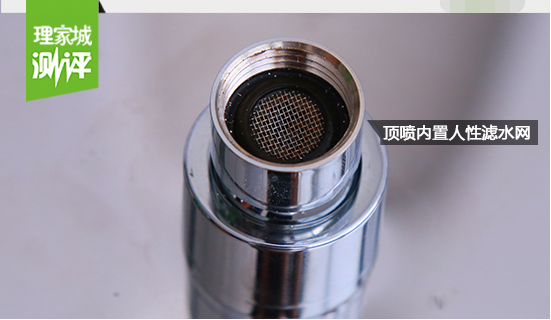小编拆开花洒顶喷、手持花洒，各衔接处都设有人性化设置滤水网，有效的防止杂物堵塞花洒头，让你的沐浴体验更舒心。

小编总评：高宝恒温花洒，让你在淋浴时告别忽冷忽热，尽情享受花洒带给你的酣畅淋漓。而一键开关方式，让你可从繁琐的传统淋浴生活方式中解脱出来，真正享受高效、便捷的一键化淋浴体验。喜欢的亲们赶紧入手吧。

`声明：本文由入驻焦点开放平台的作者撰写，除焦点官方账号外，观点仅代表作者本人，不代表焦点立场错误信息举报电话： 400-099-0099，邮箱：jubao@vip.sohu.com，或点此进行意见反馈，或点此进行举报投诉。`A B C D E F G H J K L M N P Q R S T W X Y Z
A - B - C - D - E
• A
• 鞍山
• 安庆
• 安阳
• 安顺
• 安康
• 澳门
• B
• 北京
• 保定
• 包头
• 巴彦淖尔
• 本溪
• 蚌埠
• 亳州
• 滨州
• 北海
• 百色
• 巴中
• 毕节
• 保山
• 宝鸡
• 白银
• 巴州
• C
• 承德
• 沧州
• 长治
• 赤峰
• 朝阳
• 长春
• 常州
• 滁州
• 池州
• 长沙
• 常德
• 郴州
• 潮州
• 崇左
• 重庆
• 成都
• 楚雄
• 昌都
• 慈溪
• 常熟
• D
• 大同
• 大连
• 丹东
• 大庆
• 东营
• 德州
• 东莞
• 德阳
• 达州
• 大理
• 德宏
• 定西
• 儋州
• 东平
• E
• 鄂尔多斯
• 鄂州
• 恩施
F - G - H - I - J
• F
• 抚顺
• 阜新
• 阜阳
• 福州
• 抚州
• 佛山
• 防城港
• G
• 赣州
• 广州
• 桂林
• 贵港
• 广元
• 广安
• 贵阳
• 固原
• H
• 邯郸
• 衡水
• 呼和浩特
• 呼伦贝尔
• 葫芦岛
• 哈尔滨
• 黑河
• 淮安
• 杭州
• 湖州
• 合肥
• 淮南
• 淮北
• 黄山
• 菏泽
• 鹤壁
• 黄石
• 黄冈
• 衡阳
• 怀化
• 惠州
• 河源
• 贺州
• 河池
• 海口
• 红河
• 汉中
• 海东
• I
• J
• 晋中
• 锦州
• 吉林
• 鸡西
• 佳木斯
• 嘉兴
• 金华
• 景德镇
• 九江
• 吉安
• 济南
• 济宁
• 焦作
• 荆门
• 荆州
• 江门
• 揭阳
• 金昌
• 酒泉
• 嘉峪关
K - L - M - N - P
• K
• 开封
• 昆明
• 昆山
• L
• 廊坊
• 临汾
• 辽阳
• 连云港
• 丽水
• 六安
• 龙岩
• 莱芜
• 临沂
• 聊城
• 洛阳
• 漯河
• 娄底
• 柳州
• 来宾
• 泸州
• 乐山
• 六盘水
• 丽江
• 临沧
• 拉萨
• 林芝
• 兰州
• 陇南
• M
• 牡丹江
• 马鞍山
• 茂名
• 梅州
• 绵阳
• 眉山
• N
• 南京
• 南通
• 宁波
• 南平
• 宁德
• 南昌
• 南阳
• 南宁
• 内江
• 南充
• P
• 盘锦
• 莆田
• 平顶山
• 濮阳
• 攀枝花
• 普洱
• 平凉
Q - R - S - T - W
• Q
• 秦皇岛
• 齐齐哈尔
• 衢州
• 泉州
• 青岛
• 清远
• 钦州
• 黔南
• 曲靖
• 庆阳
• R
• 日照
• 日喀则
• S
• 石家庄
• 沈阳
• 双鸭山
• 绥化
• 上海
• 苏州
• 宿迁
• 绍兴
• 宿州
• 三明
• 上饶
• 三门峡
• 商丘
• 十堰
• 随州
• 邵阳
• 韶关
• 深圳
• 汕头
• 汕尾
• 三亚
• 三沙
• 遂宁
• 山南
• 商洛
• 石嘴山
• T
• 天津
• 唐山
• 太原
• 通辽
• 铁岭
• 泰州
• 台州
• 铜陵
• 泰安
• 铜仁
• 铜川
• 天水
• 天门
• W
• 乌海
• 乌兰察布
• 无锡
• 温州
• 芜湖
• 潍坊
• 威海
• 武汉
• 梧州
• 渭南
• 武威
• 吴忠
• 乌鲁木齐
X - Y - Z
• X
• 邢台
• 徐州
• 宣城
• 厦门
• 新乡
• 许昌
• 信阳
• 襄阳
• 孝感
• 咸宁
• 湘潭
• 湘西
• 西双版纳
• 西安
• 咸阳
• 西宁
• 仙桃
• 西昌
• Y
• 运城
• 营口
• 盐城
• 扬州
• 鹰潭
• 宜春
• 烟台
• 宜昌
• 岳阳
• 益阳
• 永州
• 阳江
• 云浮
• 玉林
• 宜宾
• 雅安
• 玉溪
• 延安
• 榆林
• 银川
• Z
• 张家口
• 镇江
• 舟山
• 漳州
• 淄博
• 枣庄
• 郑州
• 周口
• 驻马店
• 株洲
• 张家界
• 珠海
• 湛江
• 肇庆
• 中山
• 自贡
• 资阳
• 遵义
• 昭通
• 张掖
• 中卫

1室1厅1厨1卫1阳台

1
2
3
4
5

0
1
2

1

1

0
1
2
3报名成功，资料已提交审核A B C D E F G H J K L M N P Q R S T W X Y Z
A - B - C - D - E
• A
• 鞍山
• 安庆
• 安阳
• 安顺
• 安康
• 澳门
• B
• 北京
• 保定
• 包头
• 巴彦淖尔
• 本溪
• 蚌埠
• 亳州
• 滨州
• 北海
• 百色
• 巴中
• 毕节
• 保山
• 宝鸡
• 白银
• 巴州
• C
• 承德
• 沧州
• 长治
• 赤峰
• 朝阳
• 长春
• 常州
• 滁州
• 池州
• 长沙
• 常德
• 郴州
• 潮州
• 崇左
• 重庆
• 成都
• 楚雄
• 昌都
• 慈溪
• 常熟
• D
• 大同
• 大连
• 丹东
• 大庆
• 东营
• 德州
• 东莞
• 德阳
• 达州
• 大理
• 德宏
• 定西
• 儋州
• 东平
• E
• 鄂尔多斯
• 鄂州
• 恩施
F - G - H - I - J
• F
• 抚顺
• 阜新
• 阜阳
• 福州
• 抚州
• 佛山
• 防城港
• G
• 赣州
• 广州
• 桂林
• 贵港
• 广元
• 广安
• 贵阳
• 固原
• H
• 邯郸
• 衡水
• 呼和浩特
• 呼伦贝尔
• 葫芦岛
• 哈尔滨
• 黑河
• 淮安
• 杭州
• 湖州
• 合肥
• 淮南
• 淮北
• 黄山
• 菏泽
• 鹤壁
• 黄石
• 黄冈
• 衡阳
• 怀化
• 惠州
• 河源
• 贺州
• 河池
• 海口
• 红河
• 汉中
• 海东
• I
• J
• 晋中
• 锦州
• 吉林
• 鸡西
• 佳木斯
• 嘉兴
• 金华
• 景德镇
• 九江
• 吉安
• 济南
• 济宁
• 焦作
• 荆门
• 荆州
• 江门
• 揭阳
• 金昌
• 酒泉
• 嘉峪关
K - L - M - N - P
• K
• 开封
• 昆明
• 昆山
• L
• 廊坊
• 临汾
• 辽阳
• 连云港
• 丽水
• 六安
• 龙岩
• 莱芜
• 临沂
• 聊城
• 洛阳
• 漯河
• 娄底
• 柳州
• 来宾
• 泸州
• 乐山
• 六盘水
• 丽江
• 临沧
• 拉萨
• 林芝
• 兰州
• 陇南
• M
• 牡丹江
• 马鞍山
• 茂名
• 梅州
• 绵阳
• 眉山
• N
• 南京
• 南通
• 宁波
• 南平
• 宁德
• 南昌
• 南阳
• 南宁
• 内江
• 南充
• P
• 盘锦
• 莆田
• 平顶山
• 濮阳
• 攀枝花
• 普洱
• 平凉
Q - R - S - T - W
• Q
• 秦皇岛
• 齐齐哈尔
• 衢州
• 泉州
• 青岛
• 清远
• 钦州
• 黔南
• 曲靖
• 庆阳
• R
• 日照
• 日喀则
• S
• 石家庄
• 沈阳
• 双鸭山
• 绥化
• 上海
• 苏州
• 宿迁
• 绍兴
• 宿州
• 三明
• 上饶
• 三门峡
• 商丘
• 十堰
• 随州
• 邵阳
• 韶关
• 深圳
• 汕头
• 汕尾
• 三亚
• 三沙
• 遂宁
• 山南
• 商洛
• 石嘴山
• T
• 天津
• 唐山
• 太原
• 通辽
• 铁岭
• 泰州
• 台州
• 铜陵
• 泰安
• 铜仁
• 铜川
• 天水
• 天门
• W
• 乌海
• 乌兰察布
• 无锡
• 温州
• 芜湖
• 潍坊
• 威海
• 武汉
• 梧州
• 渭南
• 武威
• 吴忠
• 乌鲁木齐
X - Y - Z
• X
• 邢台
• 徐州
• 宣城
• 厦门
• 新乡
• 许昌
• 信阳
• 襄阳
• 孝感
• 咸宁
• 湘潭
• 湘西
• 西双版纳
• 西安
• 咸阳
• 西宁
• 仙桃
• 西昌
• Y
• 运城
• 营口
• 盐城
• 扬州
• 鹰潭
• 宜春
• 烟台
• 宜昌
• 岳阳
• 益阳
• 永州
• 阳江
• 云浮
• 玉林
• 宜宾
• 雅安
• 玉溪
• 延安
• 榆林
• 银川
• Z
• 张家口
• 镇江
• 舟山
• 漳州
• 淄博
• 枣庄
• 郑州
• 周口
• 驻马店
• 株洲
• 张家界
• 珠海
• 湛江
• 肇庆
• 中山
• 自贡
• 资阳
• 遵义
• 昭通
• 张掖
• 中卫• 手机• 分享
• 设计
免费设计
• 计算器
装修计算器
• 入驻
合作入驻
• 联系
联系我们
• 置顶
返回顶部